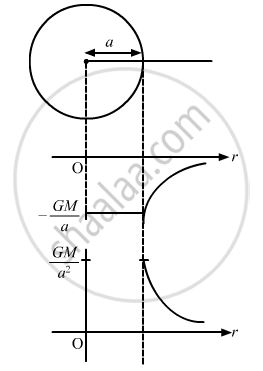Advertisement Remove all ads

# P Let V and E Be the Gravitational Potential and Gravitational Field at a Distance R from the Centre of a Uniform Spherical Shell. Consider the Following Two Statements: - Physics

MCQ

Let V and E be the gravitational potential and gravitational field at a distance r from the centre of a uniform spherical shell. Consider the following two statements :

(A) The plot of V against r is discontinuous.
(B) The plot of E against r is discontinuous.

#### Options

• Both A and B are correct.

• A is correct but B is wrong.

• B is correct but A is wrong.

• Both A and B are wrong.

Advertisement Remove all ads

#### Solution

B is correct but A is wrong.

The plot of E against r is discontinuous as gravitational field inside the spherical shell is zero (r < R). The plot of V against r is a continuous curve for a uniform spherical shell.Is there an error in this question or solution?
Advertisement Remove all ads

#### APPEARS IN

HC Verma Class 11, 12 Concepts of Physics 1
Chapter 11 Gravitation
MCQ | Q 7 | Page 224
Advertisement Remove all ads
Advertisement Remove all ads
Share
Notifications

View all notifications

Forgot password?